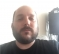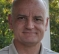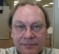## complex values in Short-time Fourier TransformStarted by 6 years ago3 replieslatest reply 6 years ago1021 views

I have an audio file as input, and I want to compute a spectrogram using STFT, manipulate magnitude and phase in the frequency domain, and then transform the signal back using the iSTFT.  Right now I am performing a test to see if I can use the STFT followed by the iSTFT with no frequency domain manipulation and get back the original signal.  The only manipulation I am doing is once I have computed the STFT, I convert the complex values from rectangular form into magnitude and phase, and then back into rectangular form.  When I iSTFT this and write out the audio file, the audio is distorted.

What is wrong the magnitude/phase calculations that I am doing?  Why doesn't W == S?

Thanks.

% convert spectrogram S to magnitude spectrogram M and phase spectrogram T
M = abs(S);
T = atan(imag(S)./real(S))

% convert back from magnitude/phase form to rectangular coordinates
newReal = M .* cos(T);
newImag = M .* sin(T);
W = newReal + j .* newImag;


[ - ]Hi,

I think that you simply need atan2(y,x) for the phase.  I may be wrong.

Remember that atan has a range of only -pi/2 -> pi/2 if you don't have the full values for real and imaginary.

Good luck.

David

[ - ]atan(0 / 1) = 0.

atan(0 / -1) = 0.

atan(1 / 1) = pi/8

atan(-1 / -1) = pi/8

etc.

What David said about atan2 -- you need to capture the whole picture on the phase, and just atan doesn't do that.

[ - ]